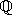CGAL::Cartesian_d<FieldNumberType>

#include <CGAL/Cartesian_d.h>

Definition

A model for Kernel_d that uses Cartesian coordinates to represent the geometric objects. In order for Cartesian_d<FieldNumberType> to model Euclidean geometry in Ed , for some mathematical field E (e.g., the rationalsor the reals), the template parameter FieldNumberType must model the mathematical field E. That is, the field operations on this number type must compute the mathematically correct results. If the number type provided as a model for FieldNumberType is only an approximation of a field (such as the built-in type double), then the geometry provided by the kernel is only an approximation of Euclidean geometry.

Kernel_d